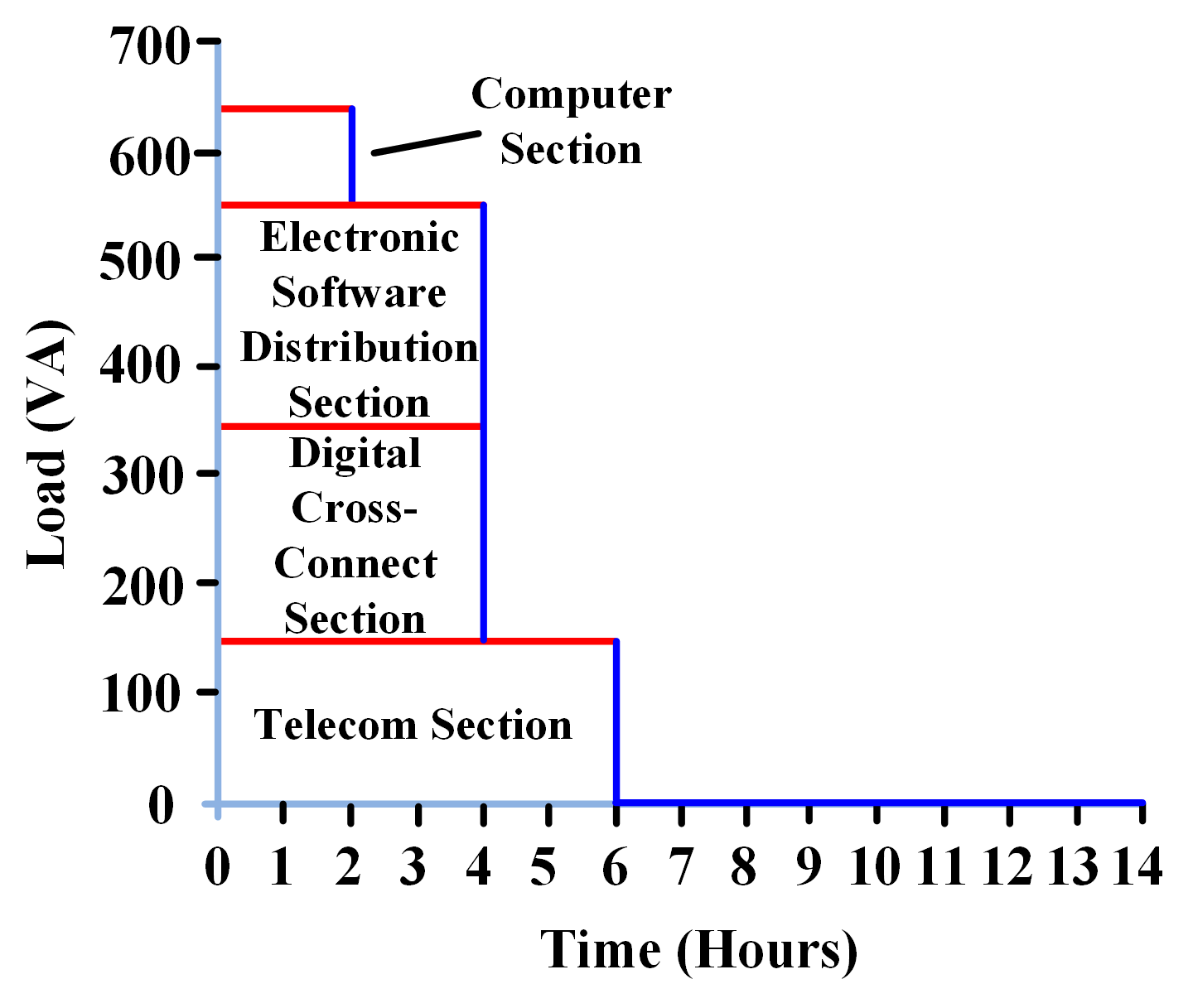# Load Profile Calculation | Solved Example

Want create site? Find Free WordPress Themes and plugins.

The load profile is an approximation of the aggregate energy required from a power system over a particular time period (e.g., years, days, hours). The load profile is demonstrated in a two-dimension graph exhibiting the instantaneous load (in Volt-Amperes) over a certain period of time and presents an easy way to visualize loads changes with time.

Approximating the energy demand is very crucial for sizing the energy storage devices such as batteries because the capacity of such devices depends on the total energy required by the connected loads. This computation is also of value for energy performance applications, where it is significant to estimate the energy usage in a system.

Calculation Approach

There are two various methods to develop load profiles:

1. 24 Hour Method

This method exhibits the average instantaneous loads over a period of 24 hours. This particular method is primarily utilized in solar PV system applications.

1. Autonomy Method

This method is primarily utilized in backup power applications such as battery systems in UPS. This method exhibits the average instantaneous loads over an autonomy (backup) time, which is the time period in which the loads need to be supplied by a backup system such as batteries.

Both techniques use the same three general steps with some minor differences:

Step 1: develop the overall connected load list

Step 2: develop the load profile

Step 3: compute the total design load and total design energy requirement

The first step is the transformation of all the collected loads into a load list.

## 24 Hour Profile Technique

In this particular method, the time period is presented in the form of “ON” and “OFF” times. These ON and OFF states are the times that the load is anticipated to be turned on and then later switched off. For certain loads that operate on the continuous basis, the ON and OFF time represent 00:00 and 23:59 respectively.

## Autonomy Technique

In this particular method, the time period is known as “autonomy” means “backup” and it represents the number of hours in which the loads need to be supplied by a backup system such as batteries during the service interruption.

Computing the Consumed Load ( VA )

Here, we can calculate the consumed apparent power of the loads in terms of Volt-Ampere. For every single load, we can compute the VA using the following formula:

${{S}_{cl}}=\frac{{{P}_{cl}}}{cos\phi \times \eta }$

Where

${{S}_{cl}}$, load consumed apparent power (VA)

${{P}_{cl}}$, load consumed active power (W)

$\eta$, load efficiency (pu)

$cos\phi$, load power factor (pu)

Step 2: Developing the Load Profile

The load profile is developed from the load list that demonstrates the load’s distribution over the period of time.

Let’s assume the loads given in the following table are based on the Autonomy (Backup) Method:

 Sections Load (VA) Autonomy (Backup) Time (Hr.) Digital Cross-Connect Section 200 4 Electronic Software DistributionSection 200 4 Telecom Section 150 6 Computer Section 90 2

The load profile is developed by heaping “energy rectangles” on top of one another. In this energy rectangle, height represents the load (VA) and the width represents the autonomy time (backup time) whereas the rectangle area represents the total load’s energy. For example, the Digital Cross-Connect Section a rectangle of width 4 (hours) and height of 200 (VA). The load profile is produced by heaping the broadest rectangles first.In the 24 Hour method, energy rectangles are developed with the time periods that a load is energized.

Step 3: Computing the Design Load & Energy Demand

The design load is the one for which all the system devices should be rated such as fuses, breakers, cables, inverters, rectifiers. The design load can be computed using the following equation:

${{S}_{des}}={{S}_{peak}}(1+{{k}_{cont}})(1+{{k}_{dm}})$

Where

${{S}_{des}}$, design load in VA

${{S}_{peak}}$, peak load in VA

${{k}_{cont}}$, contingency factor for load growth (%)

${{k}_{dm}}$, design margin (%)

It is normal to take into account future load growth in the calculations which generally ranges between 5 % and 20 % (typically somewhere between 5 and 20%). A design margin is taken into account for any inaccuracies in the load’s estimation. Generally, a design margin ranges between 10% and 15% is suggested.

Example

Let’s assume that the peak load apparent power is 640 VA. Considering a future growth of 10 % and a design margin 10%, the total design load is:

${{S}_{des}}={{S}_{peak}}(1+{{k}_{cont}})(1+{{k}_{dm}})=640\times (1+0.1)(1+0.1)=774.4VA$

Energy Demand

The design energy requirement (VAh) is utilized for energy storage devices sizing. The total design energy can be calculated by computing the area under the load profile curve. The total design energy requirement can be computed using the following equation:

${{E}_{de}}={{E}_{tle}}(1+{{k}_{cont}})(1+{{k}_{dm}})$

Where

${{E}_{de}}$, total design energy required (VAh)

${{E}_{tle}}$, total load energy (area under the load profile) in VAh

${{k}_{cont}}$, contingency factor for load growth (%)

${{k}_{dm}}$, design margin (%)

Example: From table 1 above, the total load energy is 2,680VAh. Considering a future growth of 10 % and a design margin 10%, the total design energy required is:

${{E}_{de}}=2680\times (1+0.1)(1+0.1)=3243VAh$

Did you find apk for android? You can find new Free Android Games and apps.